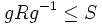# Fusion system induced by a finite group on a finite p-subgroup

## Definition

Suppose$G$ is a group (usually finite, though not necessarily so). Suppose$P$ is a subgroup of$G$ that is a finite p-group. The fusion system on$P$ induced via conjugation by$G$, which we will denote$\mathcal{F}_P(G)$, is a category defined as follows: For any$g \in G$ and$R,S \le P$ such that$gRg^{-1} \le S$, there is a morphism$\varphi:R \to S$ given by$\varphi(r) = grg^{-1}$.

Note that this category is a fusion system in the weak sense, but not necessarily a saturated fusion system, which is what many people mean when they talk of fusion system.

## Relation between the transporter system and the fusion system

Suppose$G$ is a finite group,$p$ is a prime number, and$P$ is a finite$p$-subgroup of$G$. Consider the following two categories:

• The transporter system$\mathcal{T}_P(G)$
• The fusion system$\mathcal{F}_P(G)$

The object sets of the two categories are identical, but the morphism sets differ. There is a natural "forgetful" functor from$\mathcal{T}_P(G)$ to$\mathcal{F}_P(G)$ defined as follows:

• Each object of$\mathcal{T}_P(G)$, namely, a subgroup of$P$, is sent to the same subgroup of$P$, now viewed as an object of$\mathcal{F}_P(G)$.
• The morphism set map is as follows: the element$g \in N_G(R,S)$, which is a morphism from$R$ to$S$ in$\mathcal{T}_P(G)$, gets sent to the homomorphism$\varphi:R \to S$ given by$x \mapsto gxg^{-1}$.